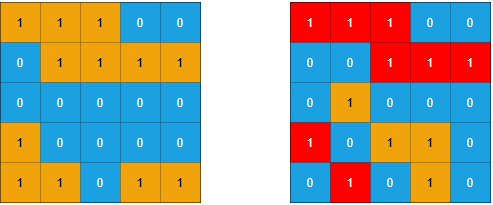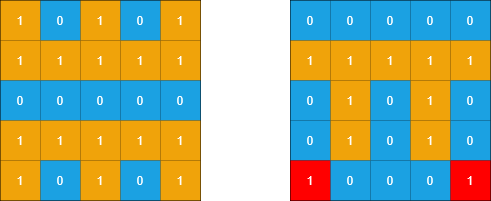# Count Sub Islands LeetCode Solution

Difficulty Level Medium
Array Depth First Search MatrixViews 171

## Problem Statement

Count Sub Islands LeetCode Solution says that grid1 and grid2 contain only `0`‘s (representing water) and `1`‘s (representing land). The island means the group of 1’s connected 4 directionally.

An island in grid2 is considered a sub-island if there is an island in grid1 that contains all the cells that make up this island in grid2.

Example 1:Input:

``` grid1 = [[1,1,1,0,0],[0,1,1,1,1],[0,0,0,0,0],[1,0,0,0,0],[1,1,0,1,1]], grid2 = [[1,1,1,0,0],[0,0,1,1,1],[0,1,0,0,0],[1,0,1,1,0],[0,1,0,1,0]]
```

Output:

``` 3
```

Explanation:

```In the picture above, the grid on the left is grid1 and the grid on the right is grid2.
The 1s colored red in grid2 are those considered to be part of a sub-island. There are three sub-islands.
```

Example 2:Input:

``` grid1 = [[1,0,1,0,1],[1,1,1,1,1],[0,0,0,0,0],[1,1,1,1,1],[1,0,1,0,1]], grid2 = [[0,0,0,0,0],[1,1,1,1,1],[0,1,0,1,0],[0,1,0,1,0],[1,0,0,0,1]]
```

Output:

``` 2
```

Explanation:

```In the picture above, the grid on the left is grid1 and the grid on the right is grid2.
The 1s colored red in grid2 are those considered to be part of a sub-island. There are two sub-islands.
```

Constraints:

• `m == grid1.length == grid2.length`
• `n == grid1[i].length == grid2[i].length`
• `1 <= m, n <= 500`
• `grid1[i][j]` and `grid2[i][j]` are either `0` or `1`.

## Approach

### Idea

1. Count Subislands, this problem can be solved through DFS on the grid.

2. We will run DFS on the second grid(grid2).

3. However, this time we also count land in matching cells in the first grid.

4. In the end, if a number of land cells match then the island in the second grid is a sub-island. We will increase our cnt variable.

## Code

### Count Sub Islands C++ Solution

```class Solution {
public:
#define ll int
ll n,m;
vector<vector<ll>>dirs={{1,0},{-1,0},{0,1},{0,-1}};
void dfs(ll x,ll y,vector<vector<ll>>&vis,vector<vector<ll>>&g1,vector<vector<ll>>&g2,ll &ans)
{
vis[x][y]=1;
ans&=g1[x][y];
for(ll i=0;i<4;i++)
{
ll ind1=x+dirs[i];
ll ind2=y+dirs[i];
if(ind1<0 or ind2<0 or ind1>=n or ind2>=m)
continue;
if(g2[ind1][ind2]==0)
continue;
if(!vis[ind1][ind2])
dfs(ind1,ind2,vis,g1,g2,ans);

}

}
int countSubIslands(vector<vector<int>>& grid1, vector<vector<int>>& grid2) {
n=grid1.size();
m=grid1.size();
vector<vector<ll>>vis(n,vector<ll>(m,0));
ll i,j,cnt=0;
for(i=0;i<n;i++)
{
for(j=0;j<m;j++)
{
if(grid2[i][j]==1 and !vis[i][j])
{
ll ans=1;
dfs(i,j,vis,grid1,grid2,ans);
if(ans==1)
{cnt++;
}
}
}
}
return cnt;
}
};```

### Count Sub Islands Java Solution

```class Solution {
int n,m;
int[] dx={1,-1,0,0};
int[] dy={0,0,1,-1};
int ans=1;
void dfs(int x,int y,int[][] vis,int[][] g1,int[][] g2)
{
vis[x][y]=1;
ans*=g1[x][y];
for(int i=0;i<4;i++)
{
int ind1=x+dx[i];
int ind2=y+dy[i];
if(ind1<0 || ind2<0 || ind1>=n || ind2>=m)
continue;
if(g2[ind1][ind2]==0)
continue;
if(vis[ind1][ind2]==0)
dfs(ind1,ind2,vis,g1,g2);

}

}

public int countSubIslands(int[][] grid1, int[][] grid2) {
n=grid1.length;
m=grid1.length;
int i,j,cnt=0;
int[][] vis = new int[n][m];
for(i=0;i<n;i++)
for(j=0;j<m;j++)
vis[i][j]=0;

for(i=0;i<n;i++)
{
for(j=0;j<m;j++)
{
if(grid2[i][j]==1 && vis[i][j]==0)
{
ans=1;
dfs(i,j,vis,grid1,grid2);
if(ans==1)
{cnt++;
}
}
}
}
return cnt;
}
}

```

## Complexity Analysis of Count Sub Islands Leetcode Solution:

### Time Complexity

The time complexity of the Count Sub Islands problem is O(N*M). Here, N*M is the dimension of the grid.

### Space Complexity

Space complexity is O(N*M). We are keeping the extra visited array while running the DFS. Here, N*M is the dimension of the grid.

Translate »